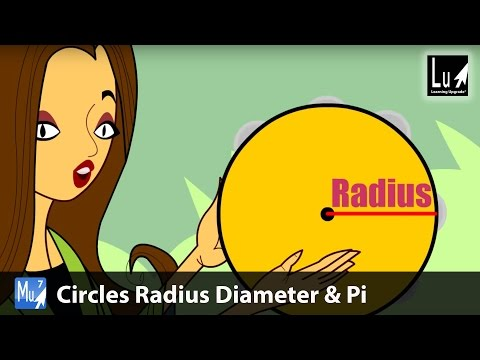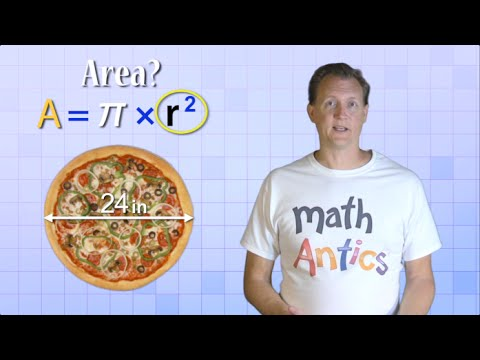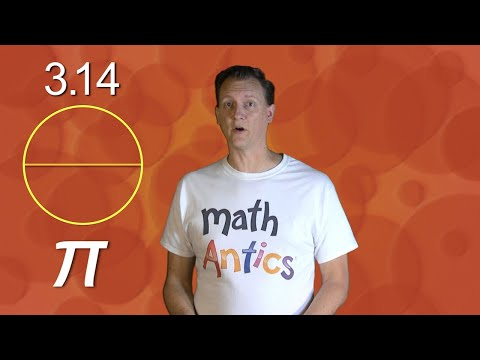## Circles

Subject: Compulsory Maths

#### Overview

The plane figure bounded by a curved line and every point of the line which is equidistance from a fixed point called the centre of the circle.The curved boundary line of a circle sis called its circumstance. The straight line is drawn from the centre of a circle to a point on its circumference is called the radius of the circle.

#### Circle and its different parts

A circle is a plane figure bounded by a curved line and every point of the line is equidistance from a fixed point called the centre of the circle.

1. Circumference of circle
The curved boundary line of a circle sis called its circumstance. The length of the circumference represents the perimeter of the circle.
The straight line is drawn from the centre of a circle to a point on its circumference is called the radius of the circle.
In the given figure, OA is the radius.
3. Chord of a circle
The straight line segment that joins any two points on the circumference of a circle is called the chords of a circle.
In the given figure, AB is the chord of the circle.
4. Diameter of a circle
The chord that passes through the centre of a circle is called the diameter of the circle. Diameter is also called the largest chord of any circle. In the given figure, CD is the diameter of a circle. The length of the diameter of a circle is two times its radius.
$\therefore$ Diameter = 2 × radius
5. Sector of a circle
The region inside a circle bounded by its two radii (plural of radius is radii) is called sector.
In the figure, the shaded region AOB is the sector.
6. Arc of a circle
The part of a curve between two given points on the curve of a circle is called an arc.
In the figure, AB is the arc.
7. Segment of a circle
The region bounded by an arc and its corresponding chord is called the segment of a circle.
In the given figure, the shaded region represents a segment.
8. Semi-circle
Half part of a circle is called a semi-circle. A diameter divides a circle into two halves and each half is the semi-circle.
In the given figure, ABC is a semi-circle.##### Things to remember
• The length of the circumference represents the perimeter of the circle.
• The length of the diameter of a circle is two times its radius.
• Half part of a circle is called a semi-circle.
• It includes every relationship which established among the people.
• There can be more than one community in a society. Community smaller than society.
• It is a network of social relationships which cannot see or touched.
• common interests and common objectives are not necessary for society.
##### Videos for Circles##### Circles Radius Diameter & Pi Song – Learn Geometry – Learning##### Math Antics - Circles, Circumference And Area##### Math Antics - Circles, What Is PI?

A circle is a plane figure bounded by a curved line and every point of the line is equidistance from a fixed point called centre of the circle.

The different parts of a circle are:

1. Circumference of a circle
3. Chord of a circle
4. Diameter of a circle
5. Sector of a circle
6. Arc of a circle
7. Segment of a circle
8. Semi-circle

The straight line drawn from the centre of a circle to a point on its circumference is called the radius of a circle whereas the chord that passes through the centre of a circle is called the diameter of a circle.

The straight line segment that joins any two points on the circumference of a circle is called the chord of a circle. However, The region bounded by an arc and its corresponding chord is called the segment of a circle.